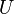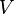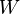# Vector space facts for kids

Kids Encyclopedia FactsAdding vectors (right side): vector a (blue) is added to vector b (red) by placing them as shown on the right. The sum, a+b, is black. Left side: the sum of two vectors makes the diagonal of a parallelogram.

A vector space is a collection of mathematical objects called vectors, along with some operations you can do on them. Two operations are defined in a vector space: addition of two vectors and multiplication of a vector with a scalar. These operations can change the size of a vector and the direction it points to. The most important thing to understand is that after you do the addition or multiplication, the result is still in the vector space; you have not changed the vector in a way that makes it not a vector anymore. A vector space is often represented using symbols such as$U$,$V$ and$W$.

More formally, a vector space is a special combination of a group and a field. The elements of the group are called vectors, and the elements of the field are called scalars. Vector spaces are important in an area of mathematics called linear algebra, an area which deals with linear functions (functions of straight lines, not curves).

A vector can be represented graphically with an arrow that has a tail and a head. To add two vectors, you place one the end of one vector at the head of the other one (see figure). The sum is the vector that goes from the tail of the first vector, to the head of the second.

Scalar multiplication means that one vector is made bigger or smaller. Scalars are just numbers: if you multiply a vector by 2, you make it twice as long. If you multiply it by 1/2, you make it half as long.

The "vectors" don't have to be vectors in the sense of things that have magnitude and direction. For example, they could be functions, matrices or simply numbers. If they obey the axioms of a vector space (a list of properties a vector space needs to satisfy), you can think of them as vectors and the theorems of linear algebra will still apply to them.

There are some combinations of vectors that are special. A minimum set of vectors that—through some combination of addition and multiplication—can reach any point in the vector space is called a basis (of that vector space). It is true that every vector space has a basis. It is also true that all bases of any one vector space have the same number of vectors in them. This is called the dimension theorem. We can then define the dimension of a vector space to be the size of its basis.

## Images for kidsVector space Facts for Kids. Kiddle Encyclopedia.